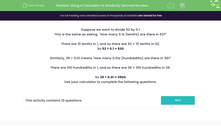# Use a Calculator to Divide by Decimal Numbers Between 0 and 1

In this worksheet, students will use calculators to divide numbers by decimals between 0 and 1.Key stage:  KS 3

Curriculum topic:   Number

Curriculum subtopic:   Use Calculators/Technology for Accuracy

Difficulty level:#### Worksheet Overview

Suppose we want to divide 52 by 0.1

This is the same as asking  "How many 0.1s (tenths) are there in 52?"

There are 10 tenths in 1, and so there are 52 × 10 tenths in 52.

So 52 ÷ 0.1 = 520

Similarly, 39 ÷ 0.01 means "How many 0.01s (hundredths) are there in 39?"

There are 100 hundredths in 1, and so there are 39 × 100 hundredths in 39.

So 39 ÷ 0.01 = 3,900

It's important for us to understand what is happening when we divide by 0.1 and 0.01, but in this activity we will be using a calculator to answer the questions.

Let's get started.

### What is EdPlace?

We're your National Curriculum aligned online education content provider helping each child succeed in English, maths and science from year 1 to GCSE. With an EdPlace account you’ll be able to track and measure progress, helping each child achieve their best. We build confidence and attainment by personalising each child’s learning at a level that suits them.

Get started